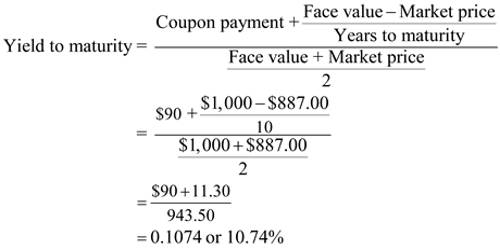# Yield to Maturity

Yield to Maturity: The yield to maturity (YTM) is that discount rate which causes the present value of the promised payment stream to equal the current price of the bond. YTM can be easily determined using a financial calculator or spreadsheet program; otherwise, one must use a trial and error process. The YTM calculation includes the assumption that you will reinvest all coupons at the same interest rate as the original bond. It asserts that the bond will be redeemed only at the end of the full maturity period. It is considered a long-term bond yield but it is expressed as an annual rate. It is expressed as an annual percentage rate.

Its calculation provides you with the total return you would receive if the bond was held through its maturity date. It is based on the coupon rate, face value, purchase price and year until maturity, calculated as:

Yield to maturity = {Coupon rate + (Face value – Purchase price/years until maturity)} / {Face value + Purchase price/2}.

This formula is used to calculate the yield on a bond based on its current price on the market.